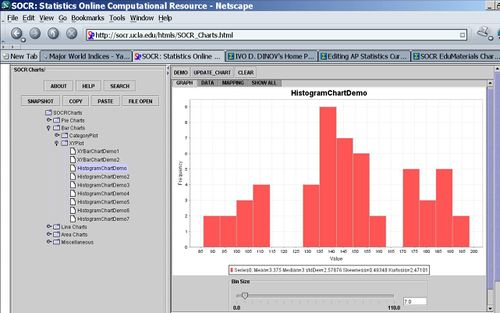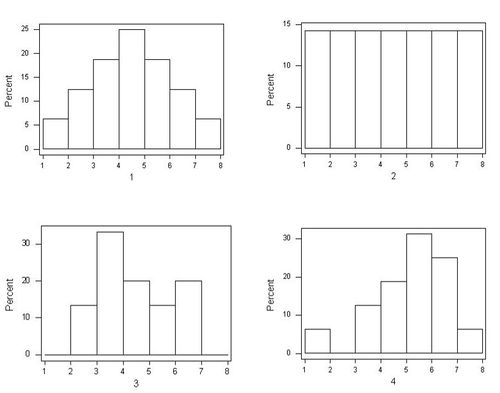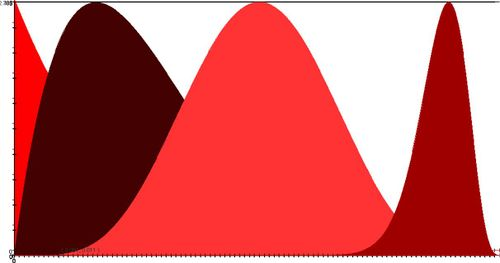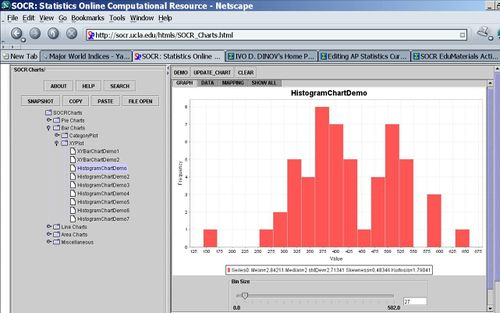## Scientific Methods for Health Sciences - Measures of Centrality, Variability and Shape

### Overview

Three main features of sample data are commonly reported as critical in understanding and interpreting the population or process that the data represent. These include Center, Spread and Shape. The main measures of centrality are Mean, Median and Mode(s). Common measures of variability include the range, the variance, the standard deviation, and mean absolute deviation. The shape of a (sample or population) distribution is an important characterization of the process and its intrinsic properties.

### Motivation

Suppose you want to synthesize the information contained in a large dataset (e.g., SOCR Data) and communicate this summary quickly and effectively to someone else. One approach is to estimate the point of centrality, the amount of dispersion and the shape of the histogram of the data and use these as concise intrinsic characteristics of the process generating the observed data.

### Theory

#### Measures of Centrality

The main measures of centrality are mean, median and mode.

Suppose we are interested in the survival times for some terminally ill patients undergoing an experimental intervention. If we conduct an ethical IRB-approved study including nine male patients and observe their survival times, as shown below. How can we determine the expected mean (center) survival time?

 74 78 106 80 68 64 60 76 98
##### Mean

The sample-mean is the arithmetic average of a finite sample of numbers. For instance, the mean of the sample $$x_1, x_2, x_3, \cdots, x_{n-1}, x_n$$. Short hand notation$\{x_{i}\}_{i=1}^n,$ is given by$\bar{x}={1\over n}\sum_{i=1}^{n}{x_{i}}.$

In the survival time example, the sample-mean is calculated as follows$\overline{y} = {1 \over 9} (74+78+106+80+68+64+60+76+98)=78.22\text{ days}.$

##### Median

The sample-median can be thought of as the point that divides a distribution in half (50/50). The following steps are used to find the sample-median:

• Arrange the data in ascending order.
• If the sample size is odd, the median is the middle value of the ordered collection.
• If the sample size is even, the median is the average of the middle two values in the ordered collection.
• For the survival time example data above, we have the following ordered data:
 60 64 68 74 76 78 80 98 106
• $$Median = 76$$.
##### Mode(s)

The modes represent the most frequently occurring values (i.e., the numbers that appear the most). The term mode is applied both to probability distributions and to collections of experimental data.

For instance, for the Hot dogs data file, there appears to be three modes for the calorie variable. This is presented by the histogram of the Calorie content of all hotdogs, shown in the image below. Note the clear separation of the calories into three distinct sub-populations; the highest points in these three sub-populations are the three modes for these data.#### Resistance

A statistic is said to be resistant if the value of the statistic is relatively unchanged by changes in a small portion of the data. Referencing the formulas for the median, mean and mode, which statistic seems to be more resistant?

If you remove the student with the long jump distance of 106 and recalculate the median and mean, which one is altered less (and is therefore more resistant)? Notice that the mean is very sensitive to outliers and atypical observations, making it less resistant than the median.

#### Resistant Mean-related Measures of Centrality

The following two sample measures of population centrality estimate resemble the calculations of the mean; however, they are much more resistant to change in the presence of outliers.

##### K-times trimmed mean

$$\bar{y}_{t,k}={1\over n-2k}\sum_{i=k+1}^{n-k}{y_{(i)}}$$, where $$k\geq 0$$ is the trim-factor (large k, yield less variant estimates of center), and $$y_{(i)}$$ are the order statistics (small to large). That is, we remove the smallest and the largest k observations from the sample, before we compute the arithmetic average.

##### Winsorized k-times mean

The Winsorized k-times mean is defined similarly by $$\bar{y}_{w,k}={1\over n}( k\times y_{(k)}+\sum_{i=k+1}^{n-k}{y_{(i)}}+k\times y_{(n-k+1)})$$, where $$k\geq 0$$ is the trim-factor and $$y_{(i)}$$ are the order statistics (small to large). In this case, before we compute the arithmetic average, we replace the k smallest and the k largest observations with the kth and (n-k)th largest observations, respectively.

#### Other Centrality Measures

The arithmetic mean answers the question: If all observations were equal, what would that value (center) have to be in order to achieve the same total? $n\times \bar{x}=\sum_{i=1}^n{x_i}$

In some situations, there is a need to think of the average in different terms, not in terms of arithmetic average.

##### Harmonic Mean

If we study speeds (velocities), the arithmetic mean is inappropriate. However, the harmonic mean (which is computed differently) gives the most intuitive answer to what the "middle" is for a process. The harmonic mean answers the question: If all the observations were equal, what would that value have to be in order to achieve the same sample sum of reciprocals?

Harmonic mean$\hat{\hat{x}}= \frac{n}{\frac{1}{x_1} + \frac{1}{x_2} + \frac{1}{x_3} + \ldots + \frac{1}{x_n}}$
##### Geometric Mean

In contrast, the geometric mean answers the question: If all the observations were equal, what would that value have to be in order to achieve the same sample product?

Geometric mean$\tilde{x}^n={\prod_{i=1}^n x_i}$
Alternatively$\tilde{x}= \exp \left( \frac{1}{n} \sum_{i=1}^n\log(x_i) \right)$

#### Measures of Variation and Dispersion

There are many measures of (population or sample) variation, including the range, the variance, the standard deviation, mean absolute deviation, etc. These are used to assess the dispersion or spread of the population.

##### Range

The range is the easiest measure of dispersion to calculate, but is probably not the best measure. The Range = max - min. For example, for the survival time data, the range is calculated by:

Range = 106 – 60 = 46.

Note that the range is only sensitive to the extreme values of a sample and ignores all other information. Therefore, two completely different distributions may have the same range.

##### Quartiles and IQR

The first quartile ($$Q_1$$) and the third quartile ($$Q_3$$) are defined values that split the dataset into bottom-25% vs. top-75% and bottom-75% vs. top-25%, respectively. Thus, the inter-quartile range (IQR), which is the difference $$Q_3 - Q_1$$, represents the central 50% of the data and can be considered as a measure of data dispersion or variation. The wider the IQR, the more variant the data.

For example, $$Q_1=68$$, $$Q_3=80$$ and $$IQR=Q_3-Q_1=12$$ for the survival time data shown above. Thus, we expect the middle half of all survival times (for that patient cohort and clinical intervention) to be between 68 and 80 days.

##### Coefficient of Variation

For a given process, the coefficient of variation ($CV$) is defined as the ratio of the standard deviation ($$\sigma$$) to the mean ($$\mu$$): $CV = \frac{\sigma}{\mu}$

Obviously, the $CV$ is well-defined for processes with well-defined first two moments (mean and variance), but also requires a non-trivial mean ($$\mu \not= 0$$). If the $CV$ is expressed as a percentage, this ratio is multiplied by 100. The sample coefficient of variation is computed mostly for data measured on a ratio scale. For instance, if a set of distances are measured, the standard deviation does not depend on whether the distances were measured in kilometers (metric) or miles. This is because changes in the particle/object's distances by 1 kilometer also changes its distance by 1 mile. However the mean distance of the data would differ in each measurement scale (as 1 mile is approximately 1.7 kilometers) and thus the coefficient of variation would differ. In general, the $CV$ may not have any meaning for data on an interval scale.

The sample-coefficient of variation is computed by plugin the sample-driven estimates of the standard deviation (sample-standard deviation, $$s$$, and the sample-average, $$\bar{x}$$). In image processing, the reciprocal of the coefficient of variation is μ/σ is called signal-to-noise-ratio ($SNR$).

##### Five-number summary

The five-number summary for a dataset is the 5-tuple $$\{min, Q_1, Q_2, Q_3, max\}$$, which contains the sample minimum, first-quartile, second-quartile (median), third-quartile, and maximum.

For example, the 5-number summary for the Long Jump data above is $(60,68,76,80,106)$.

##### Variance and Standard Deviation

The purpose of the variance and standard deviation measures is to measure the difference between each observation and the mean (i.e., dispersion). Suppose we have n > 1 observations, $$\left \{ y_1, y_2, y_3, ..., y_n \right \}$$. The deviation of the $$i^{th}$$ measurement, $$y_i$$, from the mean ($$\overline{y}$$) is defined by $$(y_i - \overline{y})$$.

Does the average of these deviations seem like a reasonable way to find an average deviation for the sample or the population? No, because the sum of all deviations is trivial:

$$\sum_{i=1}^n{(y_i - \overline{y})}=0.$$

To solve this problem, we employ different versions of the mean absolute deviation:

$${1 \over n-1}\sum_{i=1}^n{|y_i - \overline{y}|}.$$

In particular, the variance is defined as:

$${1 \over n-1}\sum_{i=1}^n{(y_i - \overline{y})^2}.$$

And the standard deviation is defined as:

$$\sqrt{{1 \over n-1}\sum_{i=1}^n{(y_i - \overline{y})^2}}.$$

For the survival time data, the standard deviation is:

$$\sqrt{{1 \over 9-1} \left \{(60-78.22)^2 + (64-78.22)^2 + (68-78.22)^2 + (74-78.22)^2 + (76-78.22)^2 + (78-78.22)^2 + (80-78.22)^2 + (106-78.22)^2 + (98-78.22)^2 \right \} } = 15.$$

### Applications

Try to pair each of the 4 samples whose numerical summaries are reported below with one of the 4 frequency plots below. Explain your answers.

 Sample Mean Median StdDev A 4.688 5.000 1.493 B 4.000 4.000 1.633 C 3.933 4.000 1.387 D 4.000 4.000 2.075#### Distribution Shape

• A distribution is unimodal if it has one mode. Unimodal distributions include:
• Bell shaped distributions (symmetric, Normal)
• Skewed right or skewed left
• We can use the mean and median to help interpret the shape of a distribution. In general, for an unimodal distribution we have these properties:
• If mean = median, the distribution is symmetric.
• If mean > median, the distribution is right skewed.
• If mean < median, the distribution is left skewed.
• Note that depending on the protocol for computing the median, these rules may be violated for certain distributions. Some examples of such violations are included in this JSE paper.
• Multimodal distributions have two or more modes. Examples of multimodal distributions are:### Software

# the R data frame "faithful" includes 272 observations on the Old Faithful geyser
# in Yellowstone National Park. Two variables are recorded: eruptions
# (how long an eruption lasted in minutes), and waiting (how long in minutes
# the geyser was quiet before that eruption)
data(faithful)
str(faithful)
attach(faithful) # attach this data so that we can easily look at the variables inside
# and "detach" it when we're done with these data!
length(waiting)
sum(is.na(waiting))
length(waiting) - sum(is.na(waiting))
sampsize = function(x) length(x) - sum(is.na(x))
sampsize(waiting)
ls()

 "AD_Associations_Data"  "avgmod.95p"            "avpred"
 "Beetle"                "cc"                    "Cement"
 "confset.95p"           "DF"                    "dsd"
 "epsilon"               "faithful"              "fm1"
 "fm2"                   "fmList"                "globmod"
 "hv"                    "MCI_Associations_Data" "ms1"
 "ms2"                   "msubset"               "myData"
 "NC_Associations_Data"  "newdata"               "newdata1"
 "Orthodont"             "p"                     "rc"
 "sampsize"              "sd"                    "test"

mean(waiting)
median(waiting)
range(waiting)
IQR(waiting)
var(waiting)
sd(waiting)

quantile(waiting, c(.75,.25), type=2)
quantile(waiting, .65)
sqrt(var(waiting)/length(waiting)) # compute the standard error of the sample mean of "waiting"
sqrt(var(wait2, na.rm=T)/length(wait2)) # to avoid calculations with "missing values" (NA's)
as.data.frame(table(waiting)) # R stores data computer-efficiently but not humanly appealing.
# To improve the display of the data we can create and print a dataframe of the data instead
stem(waiting) # create a stem-and-leaf plot of the "waiting" variable.

detach(faithful) # detach the old data
states <- as.data.frame(state.x77) # load the new data
states\$region <- state.region